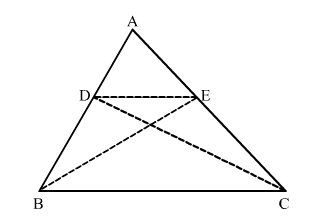# D and E are points on sides AB and AC respectively of ∆ABC such that ar(∆BCD)

Question:

D and are points on sides AB and AC respectively of ∆ABC such that ar(∆BCD) = ar(∆BCE). Prove that DE || BC.

Solution:ar(∆BCD) = ar(∆BCE)                     (Given)
We know, triangles on the same base and having equal areas lie between the same parallels.
Thus, DE || BC.